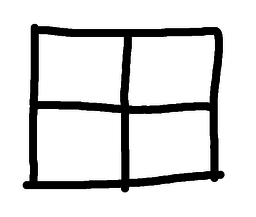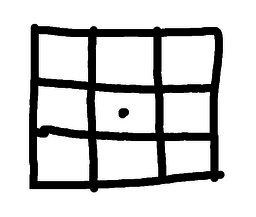# One Of My Favourite PuzzlesSubscribe!My latest posts can be found here:
Previous blog posts:

# (One of) My Favourite Puzzle(s)

It is impossible to choose a single problem as my favourite. There are so many, each with their own attractions, each with their own charms. But there is one that I solved quickly, and then found that I had only just started to scratch the surface.So we start with a simple square, and mark the centre. As a warm-up exercise, your task is to cut the square (no tricks, no gimmicks, a simple dissection) into identical pieces so that all of them touch the centre point. When I say identical, I mean identical in both size and shape, though mirror images are allowed (we mention later about forbidding them). In mathematics we say they are congruent.

As you probably realise, this isn't hard. The solution shown here is the usual first guess, but there are others. I've shown some here below.Are there any more?

Well, as it happens, yes, there are. Here are some more:So the solutions with 2 pieces and the solutions with four pieces have versions where the cuts are curved. In a sense, all the solutions with two pieces are essentially of the same form: one cut goes out from the centre to the edge, and then that cut is rotated by a half turn. If the result doesn't self-intersect, it's a solution.

Similarly, all the solutions with four pieces are of a similar form, but with quarter rotations.

So we appear to have characterised all these solutions with two or four pieces. Now we have two questions:

• Are there any other solutions with two pieces?
• (probably not)
• (but can you prove it?)

 You'll struggle, as there are four piece solutions other than as mentioned here!
• Are there any other solutions with four pieces?
• (probably not)
• (but can you prove it?)

But as yet we have no solutions with eight pieces using curved cuts. So we also get to ask:

• Are there any other solutions with eight pieces,
and where the cuts are not all straight?

• Are there any solutions with more than eight pieces?
• (Remember, the pieces are the same size and shape)

That's the warm-up puzzle, stage one.

## Warm-up, stage two ...So now that we have explored dissections where all the pieces touch the centre point, let's move on to dissections where the centre point is in the interior of a piece. At right we show one possible solution, but now we can ask more questions. We could perhaps have a five by five dissection, or a three by seven. But are these all the solutions? What are the constraints? Having found those solutions, are there any more?

And all these solutions have straight lines. Are there any solutions with curved cuts?

So:

• (a) What are all the solutions like?

and then:

• (b) Are there any solutions with curved lines?

## Warm-up, stage three ...

So we have seen solutions where all of the pieces touch the centre point, and we have seen solutions where one of the pieces touches the centre point (and hence must contain the centre point), so we wonder if there are any solutions where some pieces touch, and some pieces don't.

Specifically, are there any solutions where two or more pieces have the centre point on their boundary, but one or more pieces are not in contact with it?

This is not difficult - but remember that the pieces must all be the same size and shape. They must be congruent.

Then having found a solution, and then perhaps finding several solutions, can you find solutions with curved cuts?

So:

• (a) What are all the solutions like?
• (probably hard)

and then:

• (b) Are there any solutions with curved lines?

There are some interesting observations that can be made here that lead to solutions and potentially proofs. However, moving on ...

## Warm-up, stage four ...

So for the square we have looked at dissections with:

• All pieces touching the centre,
• One piece containing the centre, and
• Some touching, some not.

We've also asked for solutions with curved lines.

• Regular triangles?
• Regular pentagons?
• Regular hexagons?
• etc ...

Can you get solutions with all three possibilities as given above?

Are you sure?

## Finally, the puzzle ...

Consider the circle.

We can get a dissection of the circle into identical pieces that all touch the centre. Simply slice it like a pizza, and then perhaps add a little flair to use (identical) curved lines rather than the simple straight lines.

Is there is a dissection of the circle into identical pieces where the centre point is in the interior of a piece? I can tell you for free that this is an open problem - no one knows the answer for sure.

But here's the puzzle:

• Dissect a circle
• into identical pieces
• that do not all touch the centre.

# But there's more ...

When I was first presented with this problem (and without the warm-up) I quickly sketched a solution and handed it over. My companion was disappointed that I had solved it so quickly, but we moved on. Later I received an email saying that another group had a different solution. I was sceptical, but it turned out yes, there was another solution.

Mortified that I had given up so quickly I worked hard and produced an infinite family of solutions. More, for each "level" of that infinite family I had multiple solutions, starting with a few but increasing rapidly.

I had found an exploding infinite collection of solutions!

And then there was my original.

Then Joel Haddley found another solution. Working together we then generalised that into an infinite family. This was a "linear" family, but even so, an infinite one.

And then there was my original.

Sadly, we have now realised that my original solution (which in some circles (excuse the pun) is actually well known) is in fact the base case for this second infinite family.

But we thought we had completed the problem.

But no. A colleague of Joel's, Stephen Worsley, has now converted each of the solutions in this second family into a continuous family. In effect, for each solution in this second family there is a dial you can turn to create an infinite collection. So now we have an exploding countably infinite family, and a linear countably infinite family of uncountably many solutions, and we still don't know if there is a solution where the centre is in the interior.

But even if all that meant nothing, here is a final puzzle.

Can you find solutions where:

• Some edges are straight?

• All edges are curved?

• No mirror images are used?

I'm sure there remain more questions to ask, but surely this is enough for just one puzzle ...

 <<<< Prev <<<< Thinking About Recursion : >>>> Next >>>> The Independence Game ...

 You should follow me on twitter## Send us a comment ...

 You can send us a message here. It doesn't get published, it just sends us an email, and is an easy way to ask any questions, or make any comments, without having to send a separate email. So just fill in the boxes and then

 Your name : Email : Message :

# ContentsSuggest a change ( <-- What does this mean?) / Send me email